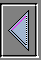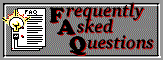## Some theory...

Introduction

LinuxThreads is a Linux library for multi-threaded programming. LinuxThreads provides kernel-level threads: threads are created with the clone() system call and all scheduling is done in the kernel. It implements the Posix 1003.1c API (Application Programming Interface) for threads and runs on any Linux system with kernel 2.0.0 or more recent, and a suitable C library.

A thread is a sequential flow of control through a program. Thus multi-threaded programming is a form of parallel programming where several threads of control are executing concurrently in the program.

Multi-threaded programming differs from Unix-style multi-processing in that all threads share the same memory space (and a few other system resources, such as file descriptors), instead of running in their own memory space as is the case with Unix processes. So a context switch between two threads in a single process is considerably cheaper than a context switch between two processes

There are two main reasons to use threads:

1. Some programs reach their best performance only expressed as several threads that communicate together (i.e. servers), rather than a single flow of instructions.

2. On a multiprocessor system, they can run in parallel on several processors, allowing a single program to divide its work between different processor. Such programs run faster than a single-thread program which can exploits only a CPU at a time.

Atomicity and volatility

Accessing the memory shared from threads require some care, because your parallel program can't access shared memory objects as they were in ordinary local memory.

Atomicity refers to the concept that an operation on an object is accomplished as an indivisible, uninterruptible, sequence. Operations on data in shared memory can occur not atomically, and, in addition of that, GCC compiler will often performs some optimizations, buffering values of shared variables in registers, avoiding the memory operations needed to ensure that all processors can see the changes on shared data.

To prevent GCC's optimizer from buffering values of shared memory objects in registers, all objects in shared memory should be declared as having types with the volatile attribute, since volatile objects reads and writes that require just one word access will occur atomically.

Locks

The load and store of the result are separate memory transactions: ++i doesn't always work to add one to a variable i in shared memory because other processors could access i between these two transactions. So, having two processes both perform ++i might only increment i by one, rather than by two.

So you need a system call that prevents a thread to work on a variable while another one is changing its value. This mechanism is implemented by the lock scheme, explained just below.
Suppose that you have two threads running a routine which change the value of a shared variable. To obtain the correct result the routine must:

• assert a lock on variable i
• modify the value of the locked variable
• remove the lock
When a lock is asserted on a variable only the thread which locked the variable can change its value. Even more the flux of the other thread is blocked on the lock assertion, since only one lock at a time is allowed for a variable. Only when the first thread remove the lock the second one can restart asserting its own lock.

Consequently using shared variables may delay memory activity from other processors, whereas ordinary references may use local cache.

## ... and some practice

Writing a multi-thread program is basically a 2 step process:

• use the pthread routines to assert locks on shared variables and generate the threads.
• create a structure for all the parameters you must pass to the thread subroutine
Let's analyze the two steps starting from a brief description of some basic pthread.h routines.

Initialize locks

One of the first actions you must accomplish is initialize all the locks. POSIX locks are declared as variables of type pthread_mutex_t; to initialize each lock you will need, call the routine:

```int pthread_mutex_init(pthread_mutex_t  *mutex,
```
as in the costruction:
```#include <pthread.h>
...
...
```
The function pthread_mutex_init initializes the mutex object pointed to by mutex according to the mutex attributes specified in mutexattr. If mutexattr is NULL, default attributes are used instead.

In the continuation is shown how to use this initialized locks.

POSIX requires the user to declare a variable of type pthread_t to identify each thread.
A thread is generated by the call to:

```
void *(*start_routine)(void *), void *arg);
```
On success, the identifier of the newly created thread is stored in the location pointed by the thread argument, and a 0 is returned. On error, a non-zero error code is returned.

To create a thread running the routine f() and pass to f() a pointer to the variable arg use:

```#include <pthread.h>
...
...
```
The routine f() must have the prototype:
```void *f(void *arg);
```
Clean termination

As the last step you need to wait for the termination of all the threads spawned before accessing the result of the routine f(). The call to:

```
```
suspends the execution of the calling thread until the thread identified by th terminates.
If thread_return is not NULL, the return value of th is stored in the location pointed to by thread_return.

Passing data to a thread routine

There are two ways to pass informations from a caller routine to a thread routine:

• Global variables
• Structures
The second one is the best choice in order to preserve the modularity of the code.
The structure must contain three levels of information; first of all informations about the shared variables and locks, second informations about all data needed by the routine; third an identification index distinguishing among threads and the number of CPU the program can exploit (making easy to provide this information at run time).

Let's inspect the first level of that structure; the information passed must be shared among every threads, so you must use pointers to the needed variables and locks. To pass a shared variable var of the type double, and its lock, the structure must contain two members:

```  double volatile *var;
```
Note the use of the volatile attribute, specifying that not pointer itself but var is volatile.

Example of parallel code

An example of program which can be easily parallelized using threads is the computation of the scalar product of two vectors.
The code is shown below with comments inserted.

```/* use gcc  -D_REENTRANT -lpthread to compile */

#include<stdio.h>

/* definition of a suitable structure */
typedef struct
{
double volatile *p_s;       /* the shared value of scalar product */
pthread_mutex_t *p_s_lock;  /* the lock for variable s */
int n;                      /* the number of the thread */
int nproc;                  /* the number of processors to exploit */
double *x;                  /* data for first vector */
double *y;                  /* data for second vector */
int l;                      /* length of vectors */
} DATA;

void *SMP_scalprod(void *arg)
{
register double localsum;
long i;
DATA D = *(DATA *)arg;

localsum = 0.0;

/* Each thread start calculating the scalar product from i = D.n
with D.n = 1, 2, ... , D.nproc.
Since there are exactly D.nproc threads the increment on i is just
D.nproc */

for(i=D.n;i<D.l;i+=D.nproc)
localsum += D.x[i]*D.y[i];

/* the thread assert the lock on s ... */

/* ... change the value of s ... */
*(D.p_s) += localsum;

/* ... and remove the lock */

return NULL;
}

#define L 9    /* dimension of vectors */

int main(int argc, char **argv)
{
void *retval;
int cpu, i;
DATA *A;
volatile double s=0;     /* the shared variable */
double x[L], y[L];

if(argc != 2)
{
printf("usage: %s  <number of CPU>\n", argv);
exit(1);
}

cpu = atoi(argv);
A = (DATA *)calloc(cpu, sizeof(DATA));

for(i=0;i<L;i++)
x[i] = y[i] = i;

/* initialize the lock variable */

for(i=0;i&lt;cpu;i++)
{
/* initialize the structure */
A[i].n = i;            /* the number of the thread */
A[i].x = x;
A[i].y = y;
A[i].l = L;
A[i].nproc = cpu;      /* the number of CPU */
A[i].p_s = &s;
A[i].p_s_lock = &s_lock;

{
fprintf(stderr, "%s: cannot make thread\n", argv);
exit(1);
}
}

for(i=0;i<cpu;i++)
{